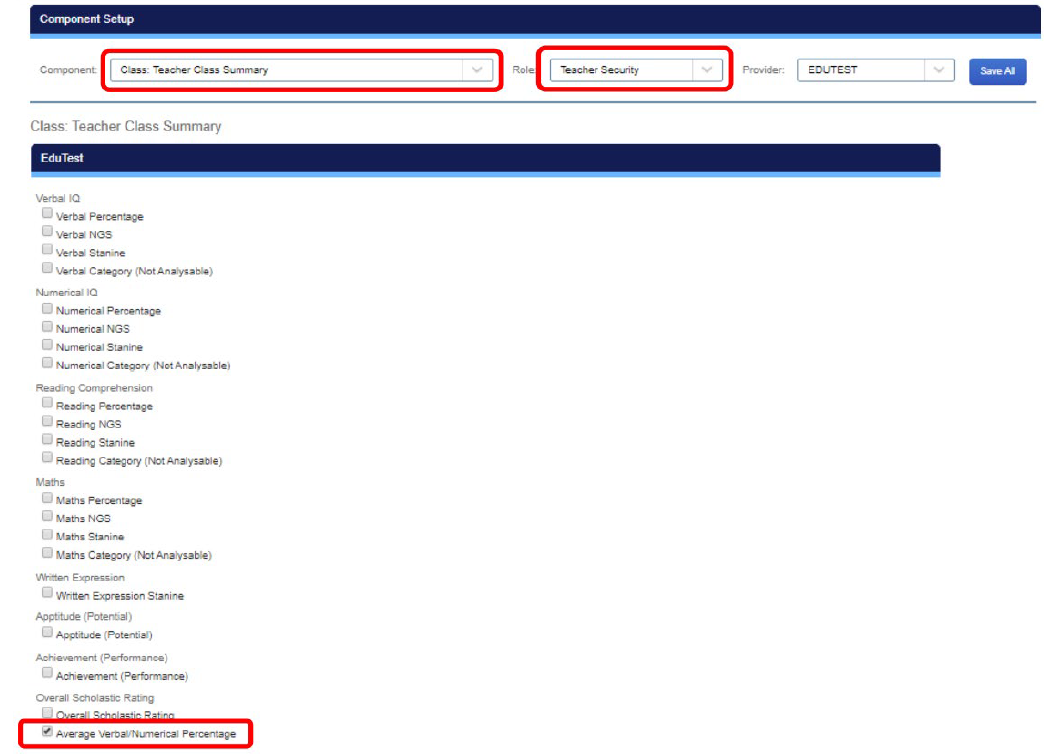# What Is a Calculated Field?

The TrackOne application has the facility to make use of calculated fields. These are data fields that ‘create’ their data by performing calculations on other data fields that the TrackOne application has stored. Common calculations that may be used include finding a percentage, average or sum of other fields. If a calculated field has been created in a data set, the ‘calculated fields’ icon will be visible next to the edit icon. The ‘calculated fields’ icon must be pressed once to recalculate all the calculated columns for the assessment item.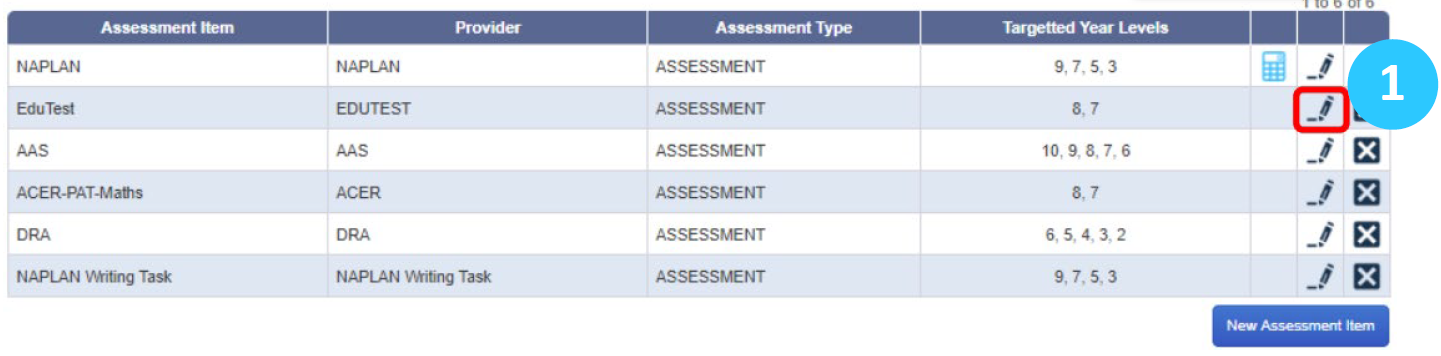1. In the TrackOne application go to Administration, then External Assessment and Assessment Items. Choose the assessment item where you want to create the calculated field and press the edit icon.
2. You will see a similar screen to the one shown below. Next choose the section where you want to create the calculated field and then press New Criteria.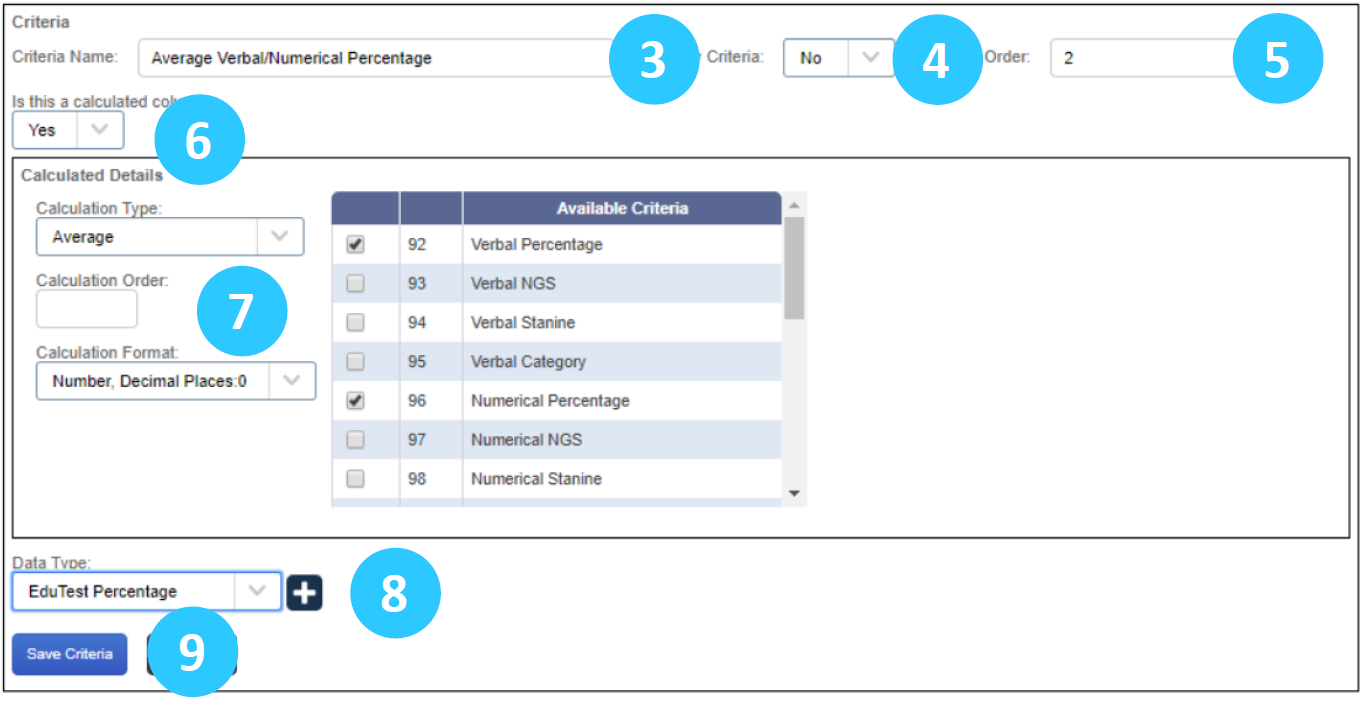3. Give your calculated field a descriptive name.
4. Choose if it is going to be your primary criteria (usually ‘No’).
5. Choose which order in the section it will be placed.
6. Set the ‘Is this a calculated column’ field to YES.
7. You then need to set the calculation type (from a drop down list), the calculation format and the fields (criteria) you want to use in the calculation.
8. Finally, set the data type for the calculated field.
9. Click on Save Criteria and you will see your new calculated field listed in the section.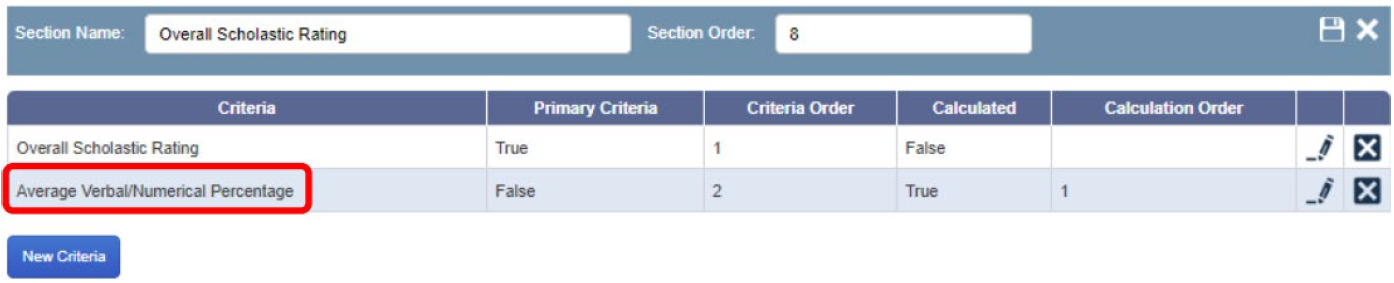10. When you go back to the Assessment Items section you will now see the calculated fields icon next to the edit icon in the Assessment item row where the calculated field was created.
11. Before you can display this calculated field, you need to press the calculated field button. This will ‘recalculate’ the data. Press OK in the dialog box.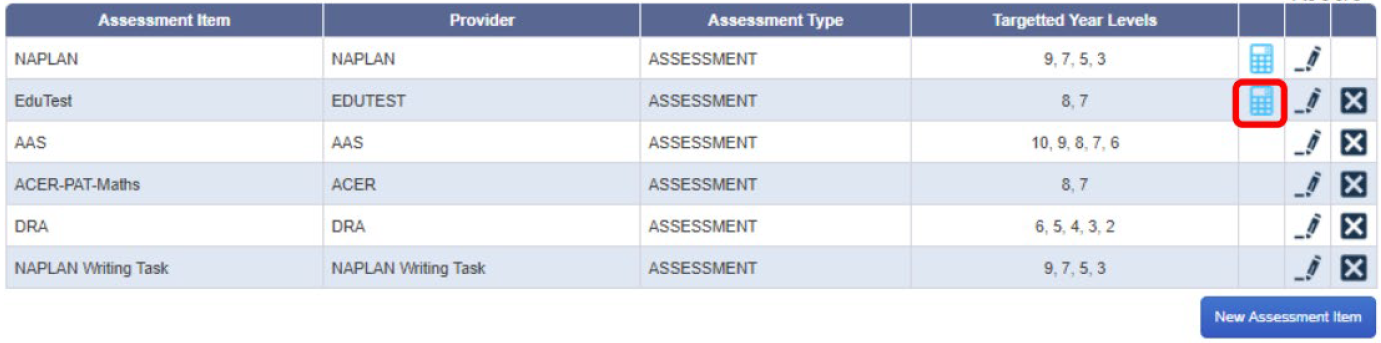You will get confirmation that the re-calculation has occurred.# Displaying the Calculated Field

To choose where your new calculated field is displayed, go to Administration, then External Assessment and Assessment Filtering. Use the Component drop down to choose ‘where’ the calculated field will be displayed and use the Role drop down to choose who will see it in that location. Check the checkbox next to your calculated field to display it. In the example below our new ‘Average Verbal/Numerical Percentage’ has been made visible on the Teacher Class Summary for all teachers.